# 3 – Shifting Shapes

 < ρℝεν | ℂσητεητs | ℕεχτ >“Science is the work of the human mind, which is destined rather to study than to know, to seek the truth rather than to find it.” – Évariste Galois

Let’s Get Physical

The image appearing above is one I drew for my previous article, Patterns patterns everywhere. However it seems to have developed a life of its own since I created it. A Google image search shows that it crops up all over the Internet whenever Group Theory is mentioned , . In the last Chapter, I defined the rules that lead to something being a Group, made the mandatory gnomic comment that these constructs were associated with symmetry and even offered some examples of symmetries that had emerged in what I had already covered. However the examples all involved numbers and the symmetries may thus have appeared somewhat theoretical.

In this chapter I’m going to introduce some Groups where the linkage to symmetry is visceral before showing the connection between these and the more abstract Groups we looked at before and – inevitably given that this set of articles is about Mathematics – returning to numbers again. I’ll start this journey with the humble equilateral triangle that is featured in my much plagiarised diagram above.

If you want to be ultra-visceral (which I am sure is a word) then by all means cut out a paper triangle of your own, label the vertices in some way and start moving it around. If you are happier dealing with an imaginary triangle, then that’s fine as well. At the end of the last Chapter, I mentioned that symmetry is related to the ways in which you can move something round while retaining the essence of what it is. So let’s talk about moving our triangle.

In order to keep track of what I am doing, I’m going to do the same as I did in my original diagram and label one vertex red, one green and one blue. There are two ways that you can move the triangle ending up with an object occupying the same space at the end. You can rotate the triangle (top row of the diagram) or you can reflect it along a line passing through any of the vertices (bottom row of the diagram). I’m going to focus first on just the rotational possibilities and a starting position with the red vertex at the top, the blue one to the left and the green one to the right.

Turning Triangles

A turn through 120° clockwise leaves the triangle in place, but the red vertex is now on the right, the blue one is at the top and the green one is to the left. From the original position, a clockwise turn of 240° means that the red vertex is to the left, the blue one to the right and the green one on top. If we reset the triangle one more time, then a rotation clockwise of 360° obviously  leaves us with the original configuration unchanged.

At this point the prose becomes somewhat cumbersome and a less prolix notation is called for. I’m going to try to provide this by describing the before and after of each move in terms of what happens to the three vertices. So: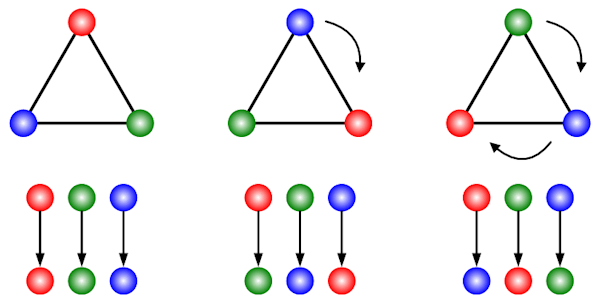There are three fairly obvious points to note.

1. A rotation of 360° (in either direction) is the same as a rotation of 0°, so we don’t need both 
2. A clockwise rotation by 240° is the same as an anticlockwise rotation of 120°, so we don’t need both
3. A rotation of 240° is the same as two rotations of 120° – while no doubt self-evident when it is described this way, we can also employ our vertex notation to show this as follows: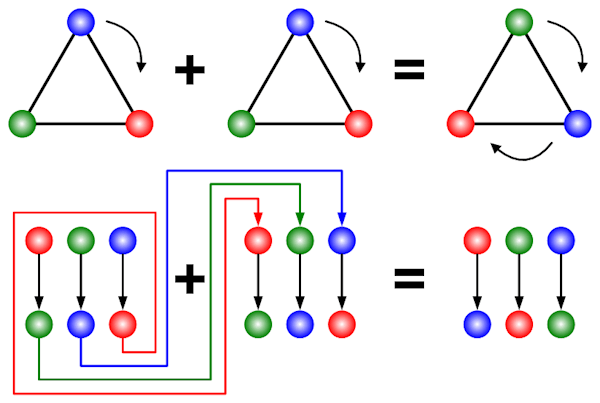To do the 120° rotation twice, we apply the vertex transformations twice, which means that the output of the first transformation is the input of the second transformation (the red, green and blue arrows trace this). If we follow the long chain of arrows on the left of the equals sign, we get the same result as following the short arrows on the right. So, using this method, we can indeed see that 120° + 120° is indeed the same as 240° 

So my assertion is that the above rotations form a Group. How do I go about proving this? Well first of all I need to recall that the devil is in the detail of how I define the set that forms the elements of the Group and the binary operator which acts upon these (again recall that a binary operator combines any two elements of the set in some way).

I’m going to define my set as the three colourful rotations (including the 360° or 0° one) I have outlined above, so our Group has as size of three. Our binary operator will be simply “do the first rotation, then do the second one”. So how do we score against our rules for something being a Group?

1. Closure

The set is small enough to just catalogue the options:

• Two 120° rotations = one 240° rotation as previously noted
• A 120° rotation followed by a 240° one (or vice versa) is a 360° (or 0°) rotation
• Two 240° rotations = one 480° rotation = one 120° rotation

So the set is closed under our binary operator

2. Identity

Clearly if we either proceed or succeed any rotation by a 0° rotation we get the same result as for the other rotation – this is also an obvious part of the Closure argument

3. Inverses

A 240° rotation followed by a 120° rotation = a 360° rotation = a 0° rotation and vice versa

4. Associativity

It’s probably self-evident that how we group up rotations makes no difference

So we do indeed have a Group. I’m going to take our previous description of which vertices map to which and change the labelling scheme noting both that we have found an identity and that a 240° rotation is the same as doing a 120° rotation twice. The result is as follows:Using the subscript labels we can write our Group as {e, r, r + r} .

At this point some bells may be ringing from the last Chapter. Recall that we encountered Modular Arithmetic (and its alternative name of Clock Arithmetic) and specifically the Group ℤ5 = {0, 1, 2, 3, 4} under the binary operator of addition Modulo 5 (remainders after dividing by 5). You may also remember that the same arguments we made proved that ℤn and the binary operator of addition Modulo n also formed a Group, where n ∈ ℕ, the Natural Numbers. What would the Modular Arithmetic Group of the same size as our triangular rotation Group look like? The answer is:

3 = {0, 1, 2}

or to look at it another way:

3 = {0, 1, 1+1}

This now begins to look very familiar and indeed the two Groups – though they arose in rather different ways – are equivalent to each other. In some sense they have the same shape, the technical term Mathematicians use is isomorphic from the Greek for “equal form” .

So we have started with a physical object – a triangle, maybe even a paper triangle if you bothered to cut one out – and ended up with one of its symmetry Groups being exactly the same shape as a purely Mathematical construct. This observation points us towards the destination of this series of articles and the deep relation between Mathematics and the Natural World.

It is finally worth noting that the geometric interpretation is called the Cyclic Group of Order 3, or C3. The Cyclic Group of Order n, or Cn, is the set of rotations of a regular polygon with n sides .

On Further Reflection…

For now let’s return to physical shapes. We could comment that the rotational symmetry Group of a square, C4, is isomorphic to ℤ4 . Instead let’s return to our humble equilateral triangle and think about the other set of symmetries, those involving reflection.

There are three lines of symmetry where a reflection round them leaves the triangle in the same place, each runs from one of the vertices to the centre of the opposing side. Here they are, together with what they do to the vertices:I’ve already labelled them up. The m stands for “mirror” and the subscript is the first letter of the vertex through which the line of reflection passes. So mg means reflection around the line going through the green vertex.

Do these reflections also form a Group (if we add in our identity element to make a Group of size 4), in the same way as the rotations do? Well let’s test our first Group property, closure, but combining two reflections, say mr and mb:We can see from the above that these two reflections combined do not equal another reflection, but instead a rotation. This rotation (r in the above example) would not be included in our candidate Group of {e, mr, mg, mb}, so this set is not closed and therefore not a Group. A lesson here in not leaping to conclusions I guess .

However, given that we have learnt that combining different reflections yields a rotation, maybe we can form a Group from both rotations and reflections. This would then be a set of six elements (including as always the transformation that does nothing – a contradiction in terms I realise) as follows:

{e, r, r2, mr, mg, mb} 

We can show that this is also a Group. I won’t offer a full proof , however the following salient facts are probably enough to at least strongly suggest this result:

1. Combining two rotations gives a rotation.
2. Combining two reflections gives a rotation.
3. Combining a reflection and a rotation and a gives a reflection.
4. The inverse of one of the rotations is the other rotation.
5. The inverse of any of the reflections is the same reflection.

The Group we have constructed has some rather interesting characteristics. One in particular stands out. Up to now all Groups we have considered have exhibited this property:

a ○ b = b ○ a

A property called commutativity, with groups fulfilling this criteria being called Commutative Groups, or Abelian Groups . However this is not part of our definition of a Group and here was have an example of a Non-commutative Group. To see this, let’s consider a rotation followed by a reflection: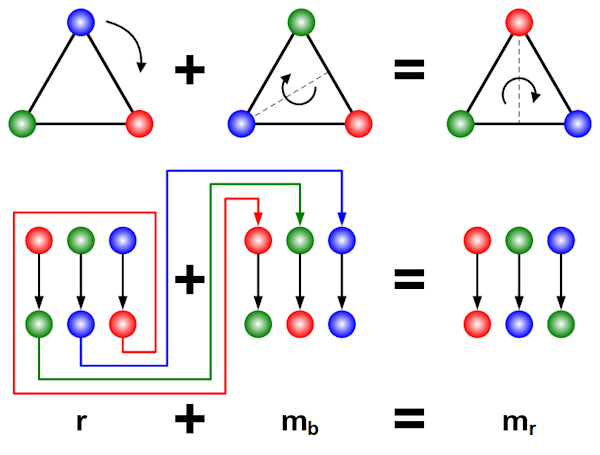and then the same reflection followed by the same rotation: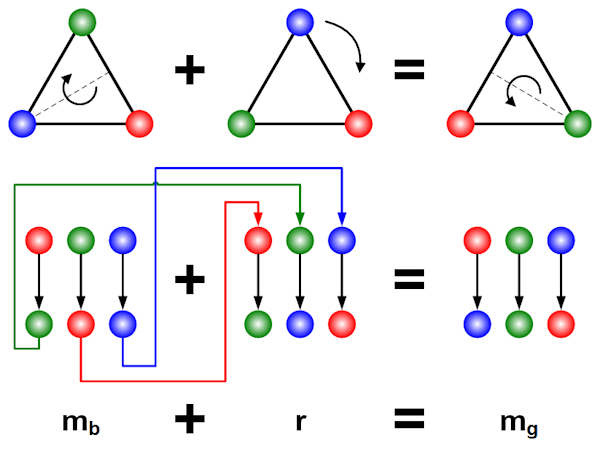So swapping the order gives us different answers and the commutative property does not hold.

The group we have been considering in this section is called the Dihedral Group of Degree 3, or D3 . The Dihedral Group of Degree n, Dn, is the set of rotations and reflections of a regular polygon with n sides. D1 and D2 are Abelian but Dn is non-Abelian for all n > 2.

Movers and Shakers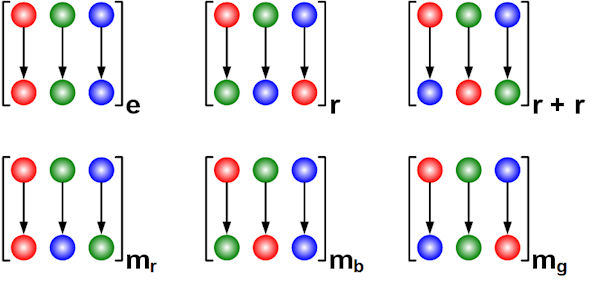In this diagram I have dropped the triangles and instead focused just on the notation that I adopted above. This is derived from how the vertices of the triangle are shifted around by different rotations and reflections. Here I am using colours for labels, so the set of things being moved around is {Red, Green, Blue}. However I could instead have picked a different set of labels, for example {a goose, a fox, a bag of grain/corn [delete as applicable]}, or letters {A, B, C}, or any other set of three distinct things. Without the geometric context it can be seen that the set of transformations appearing in the diagram have their own independent existence.

There are a number of ways in which we can reorder a set with three elements. A moment’s thought will lead to the realisation that the diagram is complete, there are no other possible rearrangements of three elements. So we can state:

all rotations and reflections of a triangle can be mapped to rearrangements of three elements

and

all rearrangements of three elements can be mapped to rotations and reflections of a triangle

This is the essence of an isomorphism. Two isomorphic Groups are essentially the same Mathematical structure dressed up in different clothes. The isomorphism is a way of translating between the two. At an essential level two isomorphic Groups are exactly the same thing.

The Mathematical name for a rearrangement like the ones we have been considering is a permutation. Is the set of six permutations shown above also a Group? Well here we can leverage the idea of isomorphism. Because these permutations are exactly the same as the rotations and reflections of the triangle (we actually generated them by looking at these transformations), and because we have already shown that the rotations and reflections of a triangle form a Group, we can definitively state that the six permutations are also form a Group; indeed it is essentially the same Group.

More generally, if two Groups are isomorphic and we establish some property of one of them, then the analogue of this property is also true in the other one. For example, if Group G is Abelian, then so are all Groups isomorphic to Group G. This provides Mathematicians with a neat trick because it may be possible to prove something very easily in one representation of a Group and very tough in another. The change of perspective offered by isomorphism means that you can prove the simpler case and be 100% confident that you have also demonstrated the harder one “for free”.

Thinking about permutations more generally, if a set has size n, then the number of ways of rearranging these is calculated as follows:

1 × 2 × 3 × … × n

The initial 1 clearly makes no difference to the calculation, but is nevertheless generally included.

This number is more tersely referred to as n factorial and written as n! In the case we have been discussing, we have:

3! = 1 × 2 × 3 = 6

The set of all Permutations of n elements forms a Group called the Permutation Group of degree n, written Sn. Here we have been dealing with S3 and have informally shown that this is isomorphic to D3. It is not true in general that Sn is isomorphic to Dn and we will meet a counter example later in the Chapter.

Turtles all the Way DownAnother observation can be made from the above discussions. We have found that one Group can happily live inside another. The Group C3 is part of the larger Group D3, we say that the former is a Subgroup of the latter. Subgroups and a particular type of Subgroup called a Normal Subgroup form a very important part of Group Theory. We will look at it in more detail in Chapter 87.

Cavorting Cubes

So we have looked at various Symmetry Groups of a two dimensional triangle, let’s add one to both of those figures and consider a three dimensional cube. Having additional dimensions to play with means that there are more symmetries potentially accessible. To put things more plainly, there are more ways in which we can spin an object around. Think about holding a cube, like a die, in your hand and the number of different ways you can twist and turn it. The image above shows all of the rotations a cube can undergo. As you will have suspected, these 24 moves (including as always our move doing nothing; the identity) form another Group. Once more this is non-Abelian, so the order in which you perform rotations can make a difference.

I’m not going to cover this Group in any detail apart from noting two things. First, we talked about S3, the Permutation Group of degree 3, earlier in this Chapter. Permutations Groups are Groups that arise out of the different ways we can reorder members of a set of given size. If we think instead about S4 then, as we mentioned above, we can calculate its size as follows:

4! = 1 × 2 × 3 × 4 = 24

It is suggestive (though by no means conclusive, as different shaped Groups can have the same number of members) that this is the same size as the the Group of rotations of a cube. In this case we can indeed show that the two Groups are isomorphic (have the same shape). One way of seeing this is to note that any rotation of the cube is a rearrangement of the four diagonals running from opposite vertices. These are the objects which are being rearranged and why the correspondence with S4 arises.

Second (and as with the triangle before), if we add in reflections as well as rotations, we get a larger Group, this time with 48 elements. This bigger Symmetry Group of the cube is called the Octahedral Symmetry Group .

The constructions we have considered in this Chapter can be generalised. Groups arise from the symmetries of more complex three dimensional objects such as dodecahedra. Indeed (following the same type of logic I applied to squares and cubes in Analogies) we can talk about the Symmetry Groups of n-dimensional objects with equal rigour to the two and three dimensional ones. Hopefully the relation between Groups and symmetry has been firmly established. In the next Chapter, we are going back to a more numerically-focused approach; one that is necessary to introduce some more Mathematics we will need later in our journey.

 Concepts Introduced in this Chapter Rotational Symmetry Regular polygons exhibit rotational symmetry, which in turn leads to the definition of a family of Groups, the Cyclic Groups. Dihedral Symmetry The same entities also have reflectional symmetry. Reflections by themselves form a Group, but the combination of rotations and reflections is also a Group. Permutation Groups Cataloguing all of the ways in which a set of n elements can be rearranged also leads to a Group. This is of size n! Or n factorial. Isomorphism It is possible to establish rigorous linkages between ostensibly different Groups to the extent that they can be viewed as the same Mathematical structure. An example here is the equivalence between the rotational symmetries of a triangle and the Group generated by considering the addition of integers Modulo 3. Another is that the rotational Group of a cube is isomorphic to the Permutation Group of 4 elements. If two Groups are isomorphic and you establish a property for one of them, the same property holds for the other. Sub-groups Groups can contain other Groups, a subject we will return to later in this book.
 Groups Discovered in this Chapter Cn The Cyclic Group of order n, which consists of the rotations of a regular polygon with n sides. More explicitly, C3 Is the set of rotations of an equilateral triangle. Dn The Dihedral Group of degree n, which consists of the rotations and reflections of a regular polygon with n sides. Again D3 is the Symmetry Group of the triangle. Sn The Permutation Group of degree n, which is the group resulting from the ways that a set of n elements can be rearranged. S3, a group of size 6, can be shown to be isomorphic to the set of rotations and reflections of an equilateral triangle. S4, a group of size 24, can be shown to be isomorphic to the set of rotations (but not reflections, see the next entry) of a cube. Octahedral Group The set of rotations and reflections of a cube, which is isomorphic to the set of rotations and reflections of an octahedron, hence the name. A group of size 48.
 < ρℝεν | ℂσητεητs | ℕεχτ >

Chapter 3 – Notes